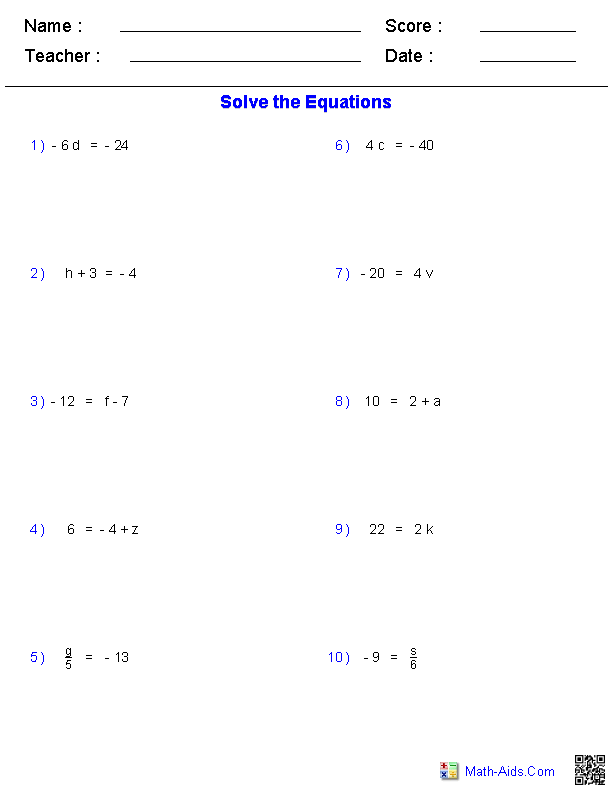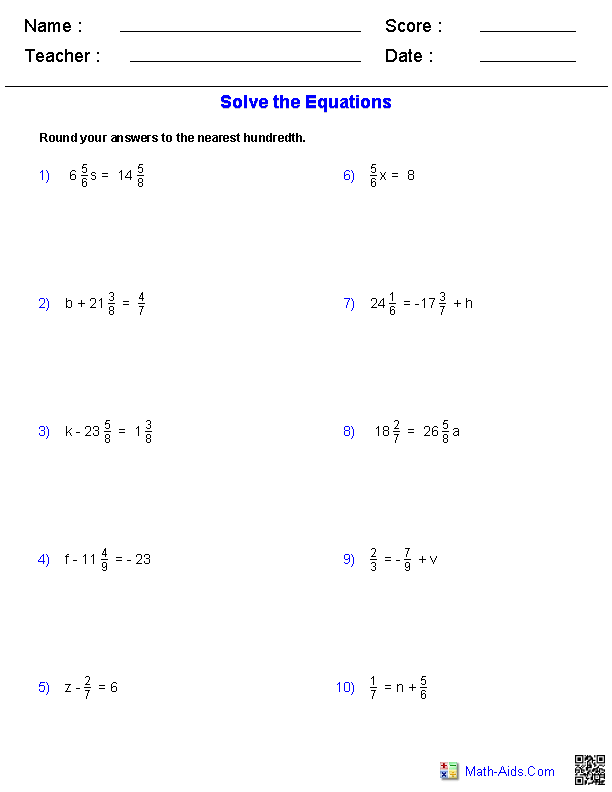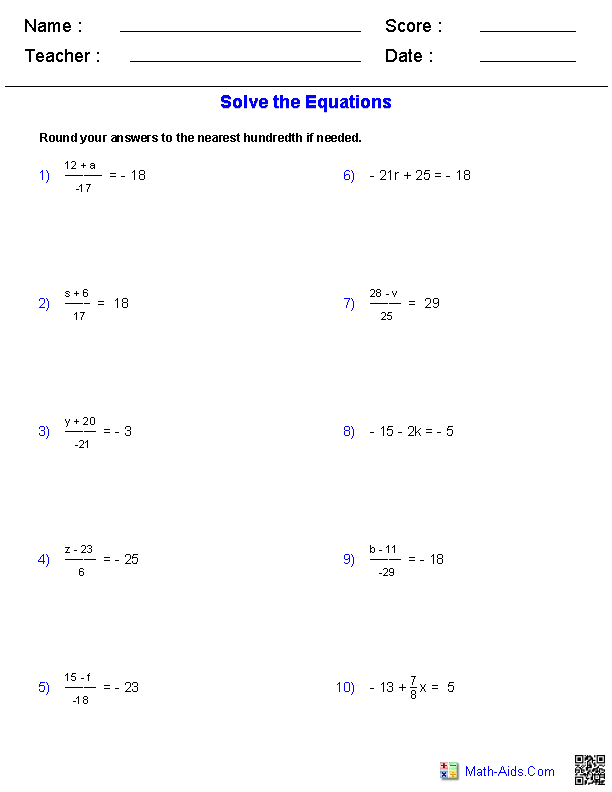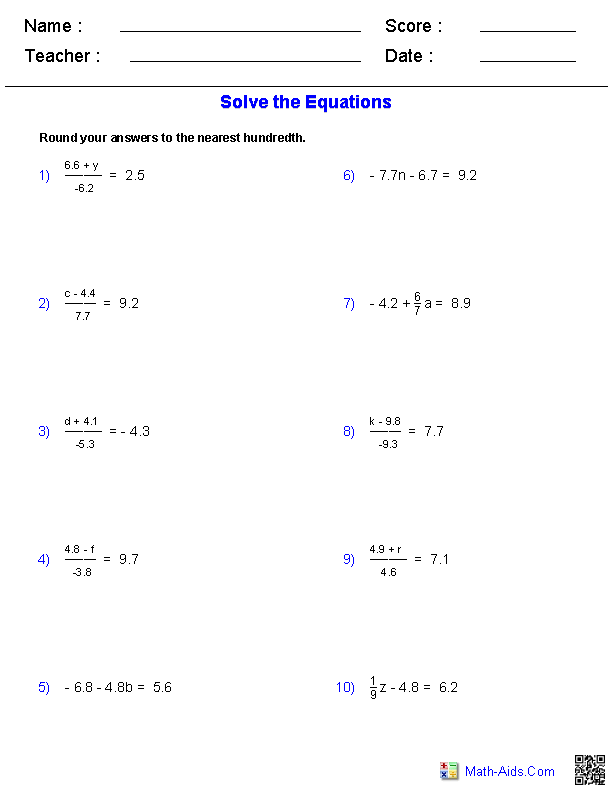#Pre-Algebra Worksheets

## Equations Worksheets

Here is a graphic preview for all of the Equations Worksheets. You can select different variables to customize these Equations Worksheets for your needs. The Equations Worksheets are randomly created and will never repeat so you have an endless supply of quality Equations Worksheets to use in the classroom or at home.

Our Equations Worksheets are free to download, easy to use, and very flexible.

These Equations Worksheets are a good resource for students in the 5th Grade through the 8th Grade.

## Quick Link for All Equations Worksheets

Click the image to be taken to that Equations Worksheets.

##### One Step EquationsIntegers Worksheets##### One Step EquationsDecimals Worksheets##### One Step EquationsFractions Worksheets##### One Step EquationWord Problems##### Two Step EquationsIntegers Worksheets##### Two Step EquationsDecimals Worksheets##### Two Step EquationWord Problems##### Multiple Step EquationsIntegers Worksheets##### Multiple Step EquationsDecimals WorksheetsRecommended Videos

## Detailed Description for All Equations Worksheets

One Step Equations Containing Integers Worksheets
These Equations Worksheets will produce one step problems containing integers. These worksheets will produce ten problems per worksheet. These Equations Worksheets are a good resource for students in the 5th Grade through the 8th Grade.

One Step Equations Containing Decimals Worksheets
These Equations Worksheets will produce one step problems containing decimals. These worksheets will produce ten problems per worksheet. These Equations Worksheets are a good resource for students in the 5th Grade through the 8th Grade.

One Step Equations Containing Fractions Worksheets
These Equations Worksheets will produce one step problems containing fractions. These worksheets will produce ten problems per worksheet. These Equations Worksheets are a good resource for students in the 5th Grade through the 8th Grade.

One Step Equation Word Problems
These Equations Worksheets will produce one step word problems. These worksheets will produce ten problems per worksheet. These Equations Worksheets are a good resource for students in the 5th Grade through the 8th Grade.

Two Step Equations Containing Integers Worksheets
These Equations Worksheets will produce two step problems containing integers. These worksheets will produce ten problems per worksheet. These Equations Worksheets are a good resource for students in the 5th Grade through the 8th Grade.

Two Step Equations Containing Decimals Worksheets
These Equations Worksheets will produce two step problems containing decimals. These worksheets will produce ten problems per worksheet. These Equations Worksheets are a good resource for students in the 5th Grade through the 8th Grade.

Two Step Equation Word Problems
These Equations Worksheets will produce two step word problems. These worksheets will produce ten problems per worksheet. These Equations Worksheets are a good resource for students in the 5th Grade through the 8th Grade.

Multiple Step Equations Containing Integers Worksheets
These Equations Worksheets will produce multiple step problems containing integers. These worksheets will produce ten problems per worksheet. These Equations Worksheets are a good resource for students in the 5th Grade through the 8th Grade.

Multiple Step Equations Containing Decimals Worksheets
These Equations Worksheets will produce multiple step problems containing decimals. These worksheets will produce ten problems per worksheet. These Equations Worksheets are a good resource for students in the 5th Grade through the 8th Grade.